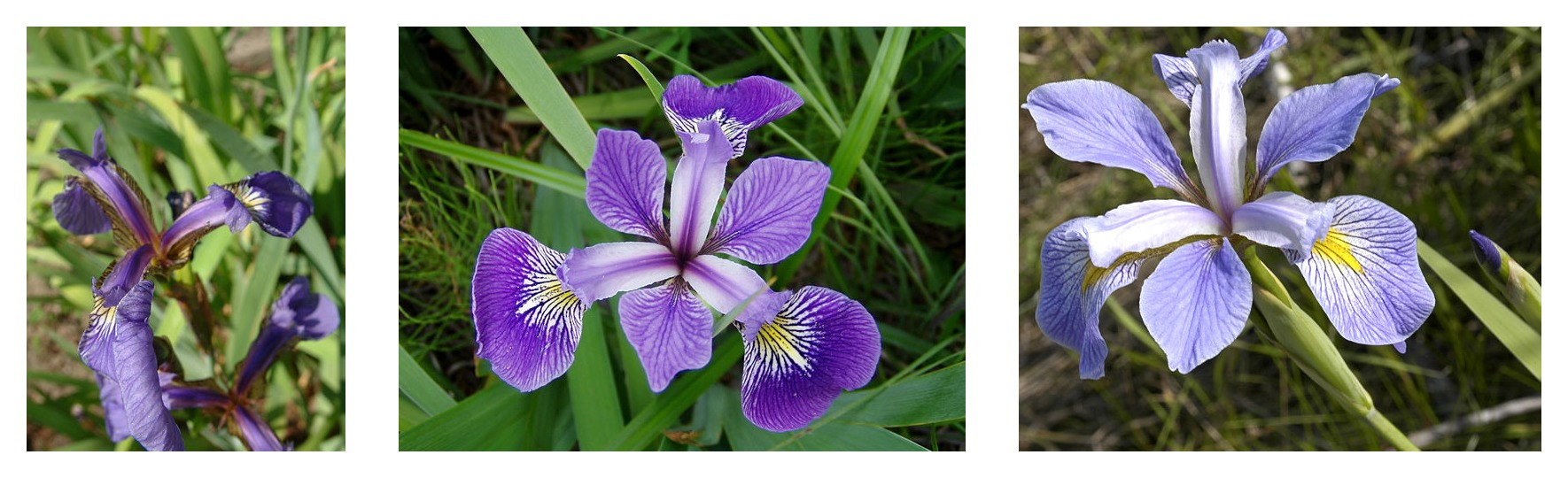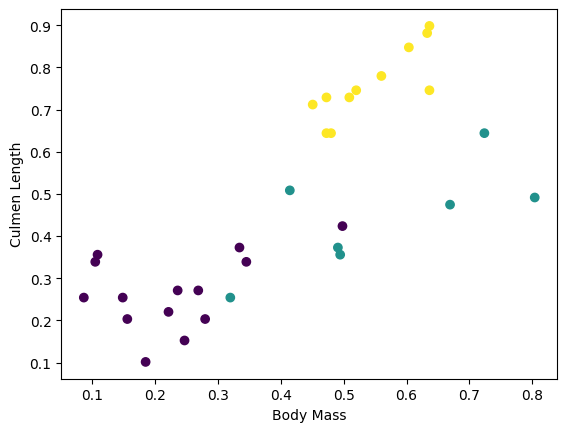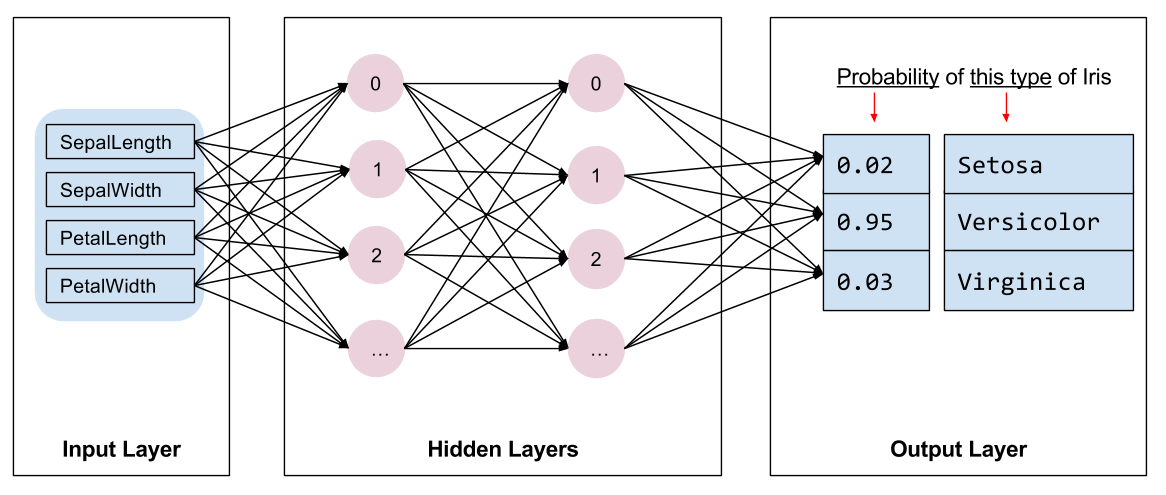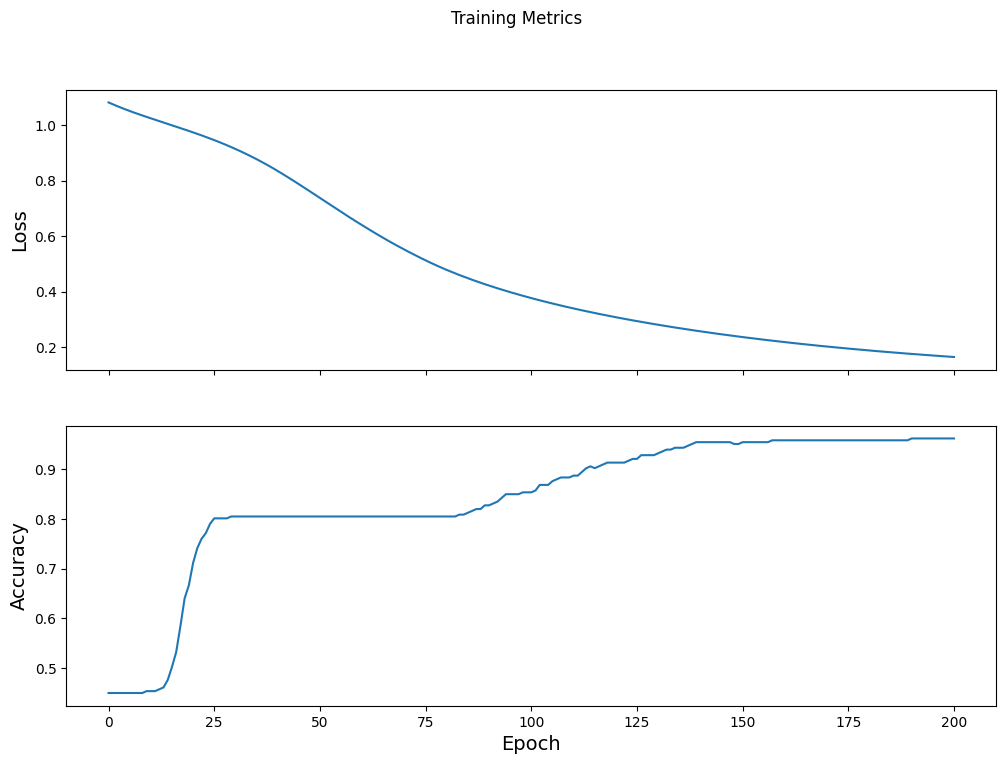TF 2.0 is out! Get hands-on practice at TF World, Oct 28-31. Use code TF20 for 20% off select passes.

自定义训练: 演示

TensorFlow 编程

1. 数据集的导入与解析
2. 选择模型类型
3. 对模型进行训练
4. 评估模型效果
5. 使用训练过的模型进行预测

环境的搭建

配置导入

from __future__ import absolute_import, division, print_function, unicode_literals

import os
import matplotlib.pyplot as plt
import tensorflow as tf
print("TensorFlow version: {}".format(tf.__version__))
print("Eager execution: {}".format(tf.executing_eagerly()))
TensorFlow version: 2.0.0-rc2
Eager execution: True

鸢尾花分类问题

• 山鸢尾
• 维吉尼亚鸢尾
• 变色鸢尾Figure 1. 山鸢尾 (by Radomil, CC BY-SA 3.0), 变色鸢尾, (by Dlanglois, CC BY-SA 3.0), and 维吉尼亚鸢尾 (by Frank Mayfield, CC BY-SA 2.0).

导入和解析训练数据集

下载数据集

train_dataset_fp = tf.keras.utils.get_file(fname=os.path.basename(train_dataset_url),
origin=train_dataset_url)

print("Local copy of the dataset file: {}".format(train_dataset_fp))
8192/2194 [================================================================================================================] - 0s 0us/step
Local copy of the dataset file: /home/kbuilder/.keras/datasets/iris_training.csv

检查数据

120,4,setosa,versicolor,virginica
6.4,2.8,5.6,2.2,2
5.0,2.3,3.3,1.0,1
4.9,2.5,4.5,1.7,2
4.9,3.1,1.5,0.1,0

• 共有 120 个样本。每个样本都有四个特征和一个标签名称，标签名称有三种可能。
• 后面的行是数据记录，每个样本各占一行，其中：
• 前四个字段是特征: 这四个字段代表的是样本的特点。在此数据集中，这些字段存储的是代表花卉测量值的浮点数。
• 最后一列是标签:即我们想要预测的值。对于此数据集，该值为 0、1 或 2 中的某个整数值（每个值分别对应一个花卉名称）。

# CSV文件中列的顺序
column_names = ['sepal_length', 'sepal_width', 'petal_length', 'petal_width', 'species']

feature_names = column_names[:-1]
label_name = column_names[-1]

print("Features: {}".format(feature_names))
print("Label: {}".format(label_name))
Features: ['sepal_length', 'sepal_width', 'petal_length', 'petal_width']
Label: species

• 0 : 山鸢尾
• 1 : 变色鸢尾
• 2 : 维吉尼亚鸢尾

class_names = ['Iris setosa', 'Iris versicolor', 'Iris virginica']

创建一个 tf.data.Dataset

TensorFlow的 Dataset API 可处理在向模型加载数据时遇到的许多常见情况。这是一种高阶 API ，用于读取数据并将其转换为可供训练使用的格式。如需了解详情，请参阅数据集快速入门指南

batch_size = 32

train_dataset = tf.data.experimental.make_csv_dataset(
train_dataset_fp,
batch_size,
column_names=column_names,
label_name=label_name,
num_epochs=1)
WARNING:tensorflow:From /tmpfs/src/tf_docs_env/lib/python3.5/site-packages/tensorflow_core/python/data/experimental/ops/readers.py:521: parallel_interleave (from tensorflow.python.data.experimental.ops.interleave_ops) is deprecated and will be removed in a future version.
Instructions for updating:
Use `tf.data.Dataset.interleave(map_func, cycle_length, block_length, num_parallel_calls=tf.data.experimental.AUTOTUNE)` instead. If sloppy execution is desired, use `tf.data.Options.experimental_determinstic`.

make_csv_dataset 返回一个(features, label) 对构建的 tf.data.Dataset ，其中 features 是一个字典: {'feature_name': value}

features, labels = next(iter(train_dataset))

print(features)
OrderedDict([('sepal_length', <tf.Tensor: id=68, shape=(32,), dtype=float32, numpy=
array([7. , 4.9, 5.2, 5.7, 6.1, 4.9, 5.7, 5.1, 5.1, 4.4, 5.4, 6.4, 5.8,
6.5, 4.9, 5.8, 5.2, 5.1, 6. , 6.8, 7.3, 5. , 7.7, 6.7, 7.2, 6.4,
6.6, 6.5, 7.6, 5. , 5. , 6.5], dtype=float32)>), ('sepal_width', <tf.Tensor: id=69, shape=(32,), dtype=float32, numpy=
array([3.2, 2.4, 3.4, 3.8, 2.8, 3. , 2.9, 3.8, 3.7, 2.9, 3.9, 3.2, 2.6,
3. , 3.1, 4. , 3.5, 3.8, 2.2, 3.2, 2.9, 3. , 2.8, 3.1, 3.2, 3.1,
3. , 2.8, 3. , 3.4, 3.3, 3. ], dtype=float32)>), ('petal_length', <tf.Tensor: id=66, shape=(32,), dtype=float32, numpy=
array([4.7, 3.3, 1.4, 1.7, 4.7, 1.4, 4.2, 1.9, 1.5, 1.4, 1.7, 4.5, 4. ,
5.8, 1.5, 1.2, 1.5, 1.5, 5. , 5.9, 6.3, 1.6, 6.7, 5.6, 6. , 5.5,
4.4, 4.6, 6.6, 1.5, 1.4, 5.2], dtype=float32)>), ('petal_width', <tf.Tensor: id=67, shape=(32,), dtype=float32, numpy=
array([1.4, 1. , 0.2, 0.3, 1.2, 0.2, 1.3, 0.4, 0.4, 0.2, 0.4, 1.5, 1.2,
2.2, 0.1, 0.2, 0.2, 0.3, 1.5, 2.3, 1.8, 0.2, 2. , 2.4, 1.8, 1.8,
1.4, 1.5, 2.1, 0.2, 0.2, 2. ], dtype=float32)>)])

plt.scatter(features['petal_length'],
features['sepal_length'],
c=labels,
cmap='viridis')

plt.xlabel("Petal length")
plt.ylabel("Sepal length")
plt.show()def pack_features_vector(features, labels):
"""将特征打包到一个数组中"""
features = tf.stack(list(features.values()), axis=1)
return features, labels

train_dataset = train_dataset.map(pack_features_vector)

Dataset 的特征元素被构成了形如 (batch_size, num_features) 的数组。我们来看看前几个样本:

features, labels = next(iter(train_dataset))

print(features[:5])
tf.Tensor(
[[6.2 3.4 5.4 2.3]
[7.4 2.8 6.1 1.9]
[5.  3.5 1.3 0.3]
[6.7 3.1 4.4 1.4]
[7.2 3.2 6.  1.8]], shape=(5, 4), dtype=float32)

选择模型类型

选择模型图 2. 包含特征、隐藏层和预测的神经网络

使用 Keras 创建模型

TensorFlow tf.keras API 是创建模型和层的首选方式。通过该 API，您可以轻松地构建模型并进行实验，而将所有部分连接在一起的复杂工作则由 Keras 处理。

tf.keras.Sequential 模型是层的线性堆叠。该模型的构造函数会采用一系列层实例；在本示例中，采用的是 2 个密集层（各自包含10个节点）,以及 1 个输出层（包含 3 个代表标签预测的节点。第一个层的 input_shape 参数对应该数据集中的特征数量，它是一项必需参数：

model = tf.keras.Sequential([
tf.keras.layers.Dense(10, activation=tf.nn.relu, input_shape=(4,)),  # 需要给出输入的形式
tf.keras.layers.Dense(10, activation=tf.nn.relu),
tf.keras.layers.Dense(3)
])

使用模型

predictions = model(features)
predictions[:5]
<tf.Tensor: id=231, shape=(5, 3), dtype=float32, numpy=
array([[ 0.764444  , -1.514788  ,  4.705434  ],
[-0.01505657, -1.4119253 ,  4.742084  ],
[ 0.4466586 , -0.7942972 ,  3.0395765 ],
[ 0.22428769, -1.1655877 ,  4.2541385 ],
[ 0.06556055, -1.5437315 ,  4.723908  ]], dtype=float32)>

tf.nn.softmax(predictions[:5])
<tf.Tensor: id=236, shape=(5, 3), dtype=float32, numpy=
array([[0.01902157, 0.0019471 , 0.9790314 ],
[0.00849907, 0.00210242, 0.9893985 ],
[0.06822292, 0.01972377, 0.91205335],
[0.01739081, 0.00433216, 0.978277  ],
[0.00937544, 0.00187536, 0.98874927]], dtype=float32)>

print("Prediction: {}".format(tf.argmax(predictions, axis=1)))
print("    Labels: {}".format(labels))
Prediction: [2 2 2 2 2 2 2 2 2 2 2 2 2 2 2 2 2 2 2 2 2 2 2 2 2 2 2 2 2 2 2 2]
Labels: [2 2 0 1 2 1 1 2 1 1 2 0 0 2 1 0 0 0 2 0 0 0 1 1 2 2 2 2 2 2 0 0]

训练模型

定义损失和梯度函数

loss_object = tf.keras.losses.SparseCategoricalCrossentropy(from_logits=True)
def loss(model, x, y):
y_ = model(x)

return loss_object(y_true=y, y_pred=y_)

l = loss(model, features, labels)
print("Loss test: {}".format(l))
Loss test: 2.253187417984009

def grad(model, inputs, targets):
with tf.GradientTape() as tape:
loss_value = loss(model, inputs, targets)
return loss_value, tape.gradient(loss_value, model.trainable_variables)

创建优化器图 3. 优化算法在三维空间中随时间推移而变化的可视化效果。(来源: 斯坦福大学 CS231n 课程，MIT 许可证，Image credit: Alec Radford)

TensorFlow有许多可用于训练的优化算法。此模型使用的是 tf.train.GradientDescentOptimizer ， 它可以实现随机梯度下降法（SGD）。learning_rate 被用于设置每次迭代（向下行走）的步长。 这是一个 超参数 ，您通常需要调整此参数以获得更好的结果。

print("Step: {}, Initial Loss: {}".format(optimizer.iterations.numpy(),
loss_value.numpy()))

print("Step: {},         Loss: {}".format(optimizer.iterations.numpy(),
loss(model, features, labels).numpy()))
Step: 0, Initial Loss: 2.253187417984009
Step: 1,         Loss: 1.8874703645706177

训练循环

1. 迭代每个周期。通过一次数据集即为一个周期。
2. 在一个周期中，遍历训练 Dataset 中的每个样本，并获取样本的特征x）和标签y）。
3. 根据样本的特征进行预测，并比较预测结果和标签。衡量预测结果的不准确性，并使用所得的值计算模型的损失和梯度。
4. 使用 optimizer 更新模型的变量。
5. 跟踪一些统计信息以进行可视化。
6. 对每个周期重复执行以上步骤。

num_epochs 变量是遍历数据集集合的次数。与直觉恰恰相反的是，训练模型的时间越长，并不能保证模型就越好。num_epochs 是一个可以调整的超参数。选择正确的次数通常需要一定的经验和实验基础。

## Note: 使用相同的模型变量重新运行此单元

# 保留结果用于绘制
train_loss_results = []
train_accuracy_results = []

num_epochs = 201

for epoch in range(num_epochs):
epoch_loss_avg = tf.keras.metrics.Mean()
epoch_accuracy = tf.keras.metrics.SparseCategoricalAccuracy()

# Training loop - using batches of 32
for x, y in train_dataset:
# 优化模型

# 追踪进度
epoch_loss_avg(loss_value)  # 添加当前的 batch loss
# 比较预测标签与真实标签
epoch_accuracy(y, model(x))

# 循环结束
train_loss_results.append(epoch_loss_avg.result())
train_accuracy_results.append(epoch_accuracy.result())

if epoch % 50 == 0:
print("Epoch {:03d}: Loss: {:.3f}, Accuracy: {:.3%}".format(epoch,
epoch_loss_avg.result(),
epoch_accuracy.result()))
Epoch 000: Loss: 1.626, Accuracy: 35.000%
Epoch 050: Loss: 0.093, Accuracy: 96.667%
Epoch 100: Loss: 0.063, Accuracy: 99.167%
Epoch 150: Loss: 0.052, Accuracy: 99.167%
Epoch 200: Loss: 0.081, Accuracy: 98.333%

可视化损失函数随时间推移而变化的情况

fig, axes = plt.subplots(2, sharex=True, figsize=(12, 8))
fig.suptitle('Training Metrics')

axes.set_ylabel("Loss", fontsize=14)
axes.plot(train_loss_results)

axes.set_ylabel("Accuracy", fontsize=14)
axes.set_xlabel("Epoch", fontsize=14)
axes.plot(train_accuracy_results)
plt.show()评估模型的效果

5.93.04.31.511
6.93.15.42.122
5.13.31.70.500
6.0 3.4 4.5 1.6 12
5.52.54.01.311

建立测试数据集

test_fp = tf.keras.utils.get_file(fname=os.path.basename(test_url),
origin=test_url)
8192/573 [============================================================================================================================================================================================================================================================================================================================================================================================================================================] - 0s 0us/step
test_dataset = tf.data.experimental.make_csv_dataset(
test_fp,
batch_size,
column_names=column_names,
label_name='species',
num_epochs=1,
shuffle=False)

test_dataset = test_dataset.map(pack_features_vector)

根据测试数据集评估模型

test_accuracy = tf.keras.metrics.Accuracy()

for (x, y) in test_dataset:
logits = model(x)
prediction = tf.argmax(logits, axis=1, output_type=tf.int32)
test_accuracy(prediction, y)

print("Test set accuracy: {:.3%}".format(test_accuracy.result()))
Test set accuracy: 96.667%

tf.stack([y,prediction],axis=1)
<tf.Tensor: id=115075, shape=(30, 2), dtype=int32, numpy=
array([[1, 1],
[2, 2],
[0, 0],
[1, 1],
[1, 1],
[1, 1],
[0, 0],
[2, 1],
[1, 1],
[2, 2],
[2, 2],
[0, 0],
[2, 2],
[1, 1],
[1, 1],
[0, 0],
[1, 1],
[0, 0],
[0, 0],
[2, 2],
[0, 0],
[1, 1],
[2, 2],
[1, 1],
[1, 1],
[1, 1],
[0, 0],
[1, 1],
[2, 2],
[1, 1]], dtype=int32)>

使用经过训练的模型进行预测

• 0: 山鸢尾
• 1: 变色鸢尾
• 2: 维吉尼亚鸢尾
predict_dataset = tf.convert_to_tensor([
[5.1, 3.3, 1.7, 0.5,],
[5.9, 3.0, 4.2, 1.5,],
[6.9, 3.1, 5.4, 2.1]
])

predictions = model(predict_dataset)

for i, logits in enumerate(predictions):
class_idx = tf.argmax(logits).numpy()
p = tf.nn.softmax(logits)[class_idx]
name = class_names[class_idx]
print("Example {} prediction: {} ({:4.1f}%)".format(i, name, 100*p))
Example 0 prediction: Iris setosa (99.9%)
Example 1 prediction: Iris versicolor (99.9%)
Example 2 prediction: Iris virginica (99.1%)